Design of Efficient Linear Feedback Shift Register for BCH Encoder | OMICS International
Journal of Electrical & Electronic Systems

# Design of Efficient Linear Feedback Shift Register for BCH Encoder

Aiswarya S1*, Manikandan SK2 and Aravinth S1

1Embedded System and Technologies Velalar College of Engineering and Technology Erode, Tamilnadu, India

2Department of Electrical and Electronics Engineering Velalar College of Engineering and Technology, Erode, Tamilnadu, India

*Corresponding Author:
Aiswarya S
M.E Student
Embedded System and Technologies
Velalar College of Engineering and Technology Erode
E-mail: [email protected]

Received Date: December 08, 2014; Accepted Date: January 22, 2014; Published Date: February 10, 2015

Citation: Aiswarya S, Manikandan SK, Aravinth S (2015) Design of Efficient Linear Feedback Shift Register for BCH Encoder. J Electr Electron Syst 4:138. doi:10.4172/2332-0796.1000138

Copyright: © 2015 Aiswarya S, et al. This is an open-access article distributed under the terms of the Creative Commons Attribution License, which permits unrestricted use, distribution, and reproduction in any medium, provided the original author and source are credited.

Visit for more related articles at Journal of Electrical & Electronic Systems

#### Abstract

The sequential circuit designed was Look-Ahead Transformation based LFSR in which a hardware complexity was present and it may limit their employ in many applications. The design of efficient LFSR for BCH encoder using TePLAT (Term Preserving Look-Ahead Transformation) overcame this limitation by opening the employ of minimizing the iteration bound and hardware complexity in wide range of applications. A TePLAT convert LFSR formulation behaves in the same way to achieve much higher throughput than those of a native implementation and a Look-Ahead Transformation-based.

#### Keywords

Linear Feedback Shift Register (LFSR); Term preserving look-ahead transformation iteration (TePLAT) bound; Loop unrolling; Look-Ahead transformation

#### Introduction

Linear Feedback Shift Register is used to generate test vectors. It uses feedback and modifies itself on every rising edge of clock. LFSR algorithms have found wide applications in wireless communication, including scrambling, error correction coding, encryption, testing, and random number generation. A LFSR is specified by its generator polynomial over the Galois Field GF (2). Some generator polynomials used on modern wireless communication applications are summarized in Tables 1 and 2 [1,2] respectively.

LFSR Index Generator Polynomial
1 1+X+x3
2 1+X+x4
3 1+x2+x5
4 1+X+x6
5 1+x4+x5+x6+x7
6 1+X+x5+x6+x8
7 1+x4+x9
8 1+x3+x10
9 1+x3+x4+x7+x12
10 1+x3+x16
11 1+x5+x12+x16
12 1+x5+x23
13 1+x2+x3+x7+x32
14 1+x+x2+x4+x5+x7+x8+x10+x12+x16+x22+x23 +x25+x3
15 1+x10+x33
16 1+x7+x42
17 1+x35+x42
18 1+x9+x49
19 1+x49+x52
20 1+x35+x74
21 1+x18+x29+x42+x57
+x67+x80

Table 1: Common LFSR-Polynomials in  and .

Wireless Standards Generator Polynomial
Wi -Fi 1+x4+x7
Wimax 1+x14+x15
LTE(Gold Code) 1+x28
1+x28+x29+x30+x31

Table 2: LFSR as Scrambler in SDR.

Traditionally LFSR can be implemented in hardware. But due to complexity in hardware LFSR can be implemented in software defined radios . Due to the mismatch of data types between the bit-serial operations of the LFSR and the word-based data path, it has been reported that 33 percent of CPU cycles of those for implementing an OFDM transmitter are dedicated to the scrambler operations. The software-implementation of the LFSR algorithm is also too slow to support real-time implementation of the 802.11 standard. This work will focus on efficient implementation of LFSR for BCH Encoder. The first approach aims at increasing execution.

Speed at the expense of additional special purpose hardware . These hardware units may interface with the host microprocessor via instruction set extensions or interrupt. The second approach seeks to reformulate LFSR algorithm so that inherent bit-level parallelism afforded by a word-based micro architecture may be fully exploited . Since a word may be regarded as a vector of binary bits, traditional vector zed compilation techniques such as loop unrolling  may be applied. The iteration bound is the inverse of theoretical maximum throughput rate an algorithm may achieve. Many LFSR polynomials such as those listed in Tables 1 and 2 have rather large loop bounds and hence cannot take full advantage of the benefit of unrolling. Fortunately, a look-ahead transformation (LAT)  promises to resolve this difficulty. However, LAT comes with a price: it often introduces additional operations. For LFSR, this implies LAT-transformed LFSR formulation may contain many more terms  than the original LFSR. These overhead may offset the potential benefit of applying LAT.

The main contribution of this work is on exploiting the low overhead property of term-preserving look-ahead transformation (TePLAT) which guarantees the number of terms of the transformed generator polynomial will remain unchanged. This term preserving property makes it feasible to apply TePLAT aggressively to achieve maximum throughput rate with respect to a particular micro architecture discussed in the context of parallel recurrent equations. This work provides critical implementation details such as initial conditions, experimental outcomes as well as applications to specific SDR platforms. The speedup factor varies from 1.5 to 18 depending on the structure of the generator polynomials.

#### Background and Definitions

Linear feedback shift register

LFSR is a shift register whose input bit is a linear function of its previous state. The XOR gate is used to provide feedback to the register that shifts bits from left to right. The maximum sequence contains all possible state except the “0000” state. Normally XOR gate is preferred for linear function of single bits. Thus, an LFSR is often a shift register whose input bit is driven by the exclusive-or (XOR) of some bits of the overall shift register value.

An LFSR can be specified by its generator polynomial over a Galois field GF (2)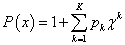(1)

Where both x and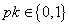, and K is the order of P(x). Each generator polynomial uniquely characterizes a linear difference equation in GF (2).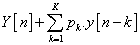(2)

Where y[n] ∈ {0, 1}, “+” is the logical XOR (exclusive-OR) operator, “.” (Multiplication) is the logical AND operator.

The beginning value of the LFSR is called the seed. Since the operation of the register is deterministic, the large number of values occurring from the register is completely determined by its present (or previous) state. Similarly, the register has a fixed number of possible states; it must finally enter a repeating cycle. However, an LFSR with a better feedback function can generate a sequence of bits which appears without any definite purpose and which has a larger cycle. The existing state may be obtained by right shifting the current state by 1 bit and filling in y[n-K-1]. Substituting n by n -1 into (2), and XO Ring y[n- K-1] on both sides, one has (note that p0 = pk= 1).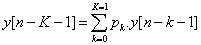(3)

The series of numbers created by a LFSR or its Exclusive-NOR counter section can be treated as binary just as Gray code or the natural binary code. The settlement of taps for feedback in an LFSR can be declared in finite field arithmetic with a polynomial of mod 2. The coefficients of the polynomial should be 1’s or 0’s. This in terms known as the reciprocal characteristic polynomial.

In the Galois configuration, when the system is clocked, the bits which are not tapped are right shifted one position unchanged. Before they are stored in the next position the taps, are XORed with the output bit. The new output bit is the next input bit. All the bits in the register shift to the right unchanged, and the input bit becomes zero only when the output bit is zero. When the output bit is one, the bits in the tap positions all flip (if they are 0, they become 1, and vice versa, finally the input bit becomes 1 only when the entire register is shifted to the right.

To generate the large number of same output, the tap order is the similar function (see above) of the order for the normal LFSR; otherwise the stream will be in reverse. It is not necessary that the LFSR’s internal should be same. The Fibonacci register and Galois register has the large number of same output as the in the initial section.

The large number of output of LFSR is based on determinism. You can predict the next state only when you know the current state and the arrangement of the XOR gates in the LFSR. It is not possible when random events occur. It is much easier to calculate the next state, with minimal-length LFSRs, as there are only an easily limited number of them for each length. The stream of output is reversible; an LFSR with similar taps will occur through the output sequence in reverse order.

A block diagram of this LFSR is depicted in Figure 1. Applying (4), one has (with K=16).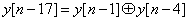(4)

Iteration bound

Recursive and adaptive digital filters are belongs to DSP algorithms which contain feedback loops, that impose an inherent essential lower bound on the achievable iterative steps or sample period. Iteration bound is the maximum of loop bound, a fundamental limit for recursive algorithms. Loop bound is the computation time divided by delay element in a loop.

Iteration bound is the inverse of theoretical maximum throughput rate an algorithm may achieve. If no delay element in the loop, then iteration bound is infinite. Clock period is lower bounded by the critical path computation time. Critical path of a DFG is the path with the longest computation time among all paths that contain zero delays.

The data dependence imposes an upper bound on how many times a loop can be unrolled to explore the inherent Inter operation parallelism. Theoretically, this kind of inter operation dependence relation is Characterized by a notion called iteration bound. Roughly, the iteration bound equals to the inverse of the number of iterations that can be unrolled into the same iteration. To increase throughput, the iteration bound must be minimized.

When the DFG is recursive, the iteration bound is the fundamental limit on the minimum sample period of a hardware implementation of the DSP program. Two algorithms to compute iteration bound are Longest Path Matrix Algorithm (LPM) and Minimum Cycle Mean (MCM). In Longest Path Matrix Algorithm (LPM) a series of matrix is constructed and the iteration bound is found by examining the diagonal elements of the matrices.

An arbitrary reference node is chosen in Gd (called this node s). The initial vector f (0) is formed by setting f(0)(s)=0 and setting the remaining nodes off(0) to infinity and find the min average length of the edge in the loop in orderto compute iteration Bound by using Minimum Cycle Mean (MCM) Method.

Loop unrolling

Loop unrolling (loop unfolding) is a well-known compiler optimization technique . It consolidates loop bodies of consecutive iterations into a single iteration to expose inherent parallelism. For example, the LFSR-10 depicted in Figure 2 can be represented as a loop (^: bitwise XOR).

However, loop unrolling cannot achieve arbitrary level of parallelism. Using LFSR-10 as an example, if one wants to unroll the loop three times, instead of two times, the following equation will need to be added into the unrolled loop body.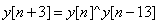(5)

However, this statement cannot be executed in the same iteration with the statement.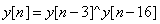(6)

Since y[n] needs to be evaluated first before it can be used to evaluate y [n+3]. This data dependence imposes an upper bound on how many times a loop can be unrolled to explore the inherent interoperation parallelism.

can be unrolled into the same iteration. To increase throughput, the iteration bound must be minimized. In Figure 2, the LFSR-10 has an iteration bound of 1/3. Hence, three successive iterations can be unrolled into the same iteration. Any path in the original DFG containing J or more delays leads to J paths with 1 or more delay in each path. Therefore, it cannot create a critical path in the J-unfolded DFG. Unfolding a DFG with iteration bound T∞ results in a J-folded DFG with iteration bound JT∞.J-unfolding of a loop with wl delays in the original DFG leads to gcd(wl,J) loops in the unfolded DFG, and each of these gcd(wl,J) loops contains wl/ gcd(wl , J) delays and J/ gcd(wl,J) copies of each node that appears in the loop.

Unfolding a DFG with iteration bound T results in a J unfolded DFG with iteration bound JT. Unfolding preserves the number of delays in a DFG. This can be stated as follows: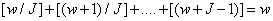(7)

Look Ahead transformation is a kind of block transformation and has the properties of block processing. In look-ahead transformation, the linear recursion is first iterated a few times to create additional concurrency. The iteration bound of this recursion is same as the original version, because the amount of computation and the number of logical delays inside the recursive loop have both doubled Look ahead Approach is also applied for Sequential Nature Decoder Algorithms. Look Ahead technique can enhance its parallel processing or block processing implementations.

Higher-order IIR digital filters can be pipelined by using clustered look-ahead or scattered look-ahead techniques. (For 1st-order IIR filters, these two look-ahead techniques reduce to the same form).In Clustered look-ahead Pipelined realizations require a linear complexity in the number of loop pipelined stages and are not always guaranteed to be stable. Scattered look-ahead can be used to derive stable pipelined IIR filters.

In this the poles of the systems will approach origin. This implies the system is more stable and limit cycle effects are reduced. The data dependency relation will be reduced. A generalized look-ahead transform in GF (2) is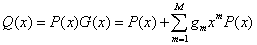(8)

#### Term Preserving Look Ahead Transformation

TePLAT of a LFSR with a generator polynomial P(x) is a LFSR with a generator polynomial Q(x)=[P(x)] ^2. Although Q(x) is a polynomial of twice the order of P(x), both of them have the same number of terms. Since this property is always true for power of two but may not necessarily hold for other exponents, we only consider power of two in TePLAT.

If the iteration bound of an LFSR is T, then the iteration bound after a TePLAT=T/2. While TePLAT promises full exploitation of bitlevel parallelism, one should not lose sight on the fact that the purpose of LFSR implementation is to generate the maximal length pseudorandom bit stream.

Each time the TePLAT is applied, the order of the transformed generator polynomial doubles. As such, twice as many bits of on-chip storage space will be needed to store the increased number of states. To fully exploit inherent bit-level parallelism, auxiliary data format conversion operations such as bit-vector packing and unpacking, and word boundary alignment will be needed (Figure 3).

If the number of states after TePLAT transformation approaches the length of the original maximal length sequence, then it may be more cost effective to simply cache the entire maximal length sequence and save all the computation. Assume that the TePLAT is repeatedly applied m times, termed as mth-level TePLAT thereafter, and then the number of bits to store the states will be 2^m*K bits. After TePLAT parallelize the LFSR to the full length of word, the number of consumed registers doubles each time we apply the technique.

LFSRs are designed for efficient implementation such as Grain stream cipher. They are generally very long and the iteration bounds are small. TePLAT result in high-order generator polynomials, more registers will be required to hold the additional bits. Hence, the memory and register-footprint of executing the LFSR algorithm should be treated as a cost function In this case; the parallelism can be achieved by simply applying loop unrolling.

In terms of cost, since LAT and TePLAT both result in highorder generator polynomials, more registers will be required to hold the additional bits. Hence, the memory and register-footprint of executing the LFSR algorithm should be treated as a cost function. The improvement does not induce any hardware overhead. However, after TePLAT parallelize the LFSR to the full length of word, the number of consumed.

The conventional LFSRs are similar to the applied loop unrolling (LU) technique. TePLAT may also be applied, with careful tradeoffs between area and throughput, to hardware-based LFSR implementation. Assume that the TePLAT is repeatedly applied m times, termed as mthlevel TePLAT thereafter, and then the number of bits to store the states will be 2^m K bits. Using above argument, one must limit m such that 2m.K ≤ 2k. After simplification, one has m ≤ [K -log2kc].

#### Simulation Result

The cycle-accurate simulator can profile convincible outcome for demonstrating this algorithm. Therefore, Texas instruments Inc., Code Composer Studio (CCS) and advanced RISC Machines Ltd. Instruction Set Simulator (ARMulator) is used.

In this work, an in-house source-to-source compiler that generates LFSR codes with TePLAT factors ranging from 2^0 to 2^8 is built. The generated codes on the corresponding simulators are simulated and determined the best TePLAT factor for the LFSR.

The best performance look-ahead transformed LFSR found based on the experiments is termed as “best” in the following results. Popular and representative processor for mobile devices such as TI-C6416 digital signal processor is used. The best look-ahead transformed LFSR’s improvement depends on the LFSR generator polynomial and the Processor architecture. A comparison of the optimization technique is provided in Figure 4.

Throughput numbers are given for all the LFSRs. The conventional LFSRs are similar to that applied loop unrolling (LU) technique.

The best look-ahead transformed LFSR’s improvement depends on the LFSR generator polynomial and the processor architecture. Our experimental results show that the best LFSR can usually be found by TePLAT factor ranging from 2^0 to 2^8. The best look-ahead transformed LFSRs can perform at most 18 * (LFSR-8) to 50 percent (LTE) faster.

In , bit manipulation unit (BMU) hardware was proposed to accelerate a communication DSP and was implemented on XILINX VirtexII. The throughput of Wifi scrambler in  is 0.6 bit/cycle. Our method can achieve 0.7 bit/cycle on ARM and 2.9 bit/cycle on TI.

If the throughput is measured using bit/sec (bps), the 180 nm DSP in  achieves throughput of 168 Mbps and our proposed framework is 280 Mbps and 1.74 Gbps on 130 nm ARM926 and 130 nm TI C6416, respectively. They are generally very long and the iteration bounds are small. In this case, the parallelism can be achieved by simply applying loop unrolling, and thus our TePLAT methods have negligible improvement.

#### Conclusion

The Design of Efficient Linear Feedback shift Register is to minimize iteration bound without introducing any additional operations. A term preserving look-ahead transformation (TePLAT) is used for efficient parallel implementation of LFSR in several applications. Compared to existing approaches there will be significant speedup performance and also the hardware utilization will be minimized. Compared to existing approaches, significant speedup has been observed in numerous simulations. TePLAT may also be applied, with careful tradeoffs between area and throughput, to hardware-based LFSR implementation.

#### References

Select your language of interest to view the total content in your interested language

### Article Usage

• Total views: 13459
• [From(publication date):
June-2015 - Feb 19, 2020]
• Breakdown by view type
• HTML page views : 9603Can't read the image? click here to refresh